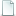## Multivariable Calculus - Math 53 - Fall 2009 - UC Berkeley Edward Frenkelmultivariable-calculus-math-53-001-fall2009-ucberkeley (25 files)Mathematics - Multivariable Calculus - Lecture 19-AjW54NziKDo.mp4 218.58MBMathematics - Multivariable Calculus - Lecture 18-Mh_QF6LoQcc.mp4 217.71MBMathematics - Multivariable Calculus - Lecture 17-ccbd0OeVbEs.mp4 223.91MBMathematics - Multivariable Calculus - Lecture 16-70oLiVKNqhU.mp4 214.27MBMathematics - Multivariable Calculus - Lecture 15-qzwwEg9Gjto.mp4 323.29MBMathematics - Multivariable Calculus - Lecture 14-Wfa5gRY8XKI.mp4 217.36MBMathematics - Multivariable Calculus - Lecture 13-BMZ0_YyNf5M.mp4 217.30MBMathematics - Multivariable Calculus - Lecture 12-7cPcutRLLXE.mp4 213.60MBMathematics - Multivariable Calculus - Lecture 11-IsZm5_HkmmQ.mp4 218.43MBMathematics - Multivariable Calculus - Lecture 10-MxPSOoKvGYY.mp4 214.14MBMathematics - Multivariable Calculus - Lecture 9-vHNDOgggNfE.mp4 320.57MBMathematics - Multivariable Calculus - Lecture 8-dD2P_9NKVSI.mp4 215.35MBMathematics - Multivariable Calculus - Lecture 7-QJRCE6FSn10.mp4 313.08MBMathematics - Multivariable Calculus - Lecture 6-NF7C36OYT_A.mp4 219.17MBMathematics - Multivariable Calculus - Lecture 5-ejejmdw9E7E.mp4 219.28MBMathematics - Multivariable Calculus - Lecture 4-fYwJcebwaA0.mp4 209.72MBMathematics - Multivariable Calculus - Lecture 3-0AXeBcfKe_A.mp4 214.39MBMathematics - Multivariable Calculus - Lecture 2-GMZAXLNiVkI.mp4 216.97MBMathematics - Multivariable Calculus - Lecture 1-cw6pHhjhKmk.mp4 217.19MBMathematics - Multivariable Calculus - Lecture 20-neuUOyHgsiE.mp4 224.18MBMathematics - Multivariable Calculus - Lecture 21-QUaKLCfOJCc.mp4 327.59MBMathematics - Multivariable Calculus - Lecture 22-U2L5Z1H140g.mp4 217.48MBMathematics - Multivariable Calculus - Lecture 23-7tHkqh8m9UM.mp4 222.23MBMathematics - Multivariable Calculus - Lecture 24-Vwky37BxB3U.mp4 220.62MBMathematics - Multivariable Calculus - Lecture 25-FZb4Xuz4hkc.mp4 204.02MB
Type: Course
Tags:

Bibtex:
```@article{,
title= {Multivariable Calculus - Math 53 - Fall 2009 - UC Berkeley},
author= {Edward Frenkel},
keywords= {},
abstract= {Math 53 - Section 1 - Multivariable Calculus
Instructor: Edward Frenkel

Lectures: TT 3:30-5:00pm, Room 155 Dwinelle

Course Control Number: 54296

Office: 819 Evans

Office Hours: TBA

Prerequisites: Math 1A, 1B.

Required Text: Stewart, Multivariable Calculus, (custom edition).

Syllabus:

Course Webpage: To be linked from http://math.berkeley.edu/~frenkel/Math53

Grading: 25% quizzes and HW, 20% each midterm, 35% final

Homework: Homework for the entire course will be assigned at the beginning of the semester, and weekly
homework will be due at the beginning of each week.

Comments: Students have to make sure that they have no scheduling conflicts with the final exam. Missing final exam means automatic Fail grade for the entire course.},
terms= {},
url= {https://web.archive.org/web/20100501160242/http://math.berkeley.edu/~frenkel/Math53},
year= {2009}
}

```

Send Feedback